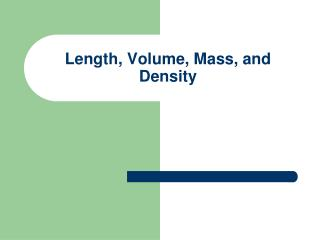DownloadDownload PresentationLength, Volume, Mass, and Density

# Length, Volume, Mass, and Density

Télécharger la présentation## Length, Volume, Mass, and Density

- - - - - - - - - - - - - - - - - - - - - - - - - - - E N D - - - - - - - - - - - - - - - - - - - - - - - - - - -
##### Presentation Transcript

1. Length, Volume, Mass, and Density

2. Length • How “long” something is • A ruler is used to measure length

3. Practice • Complete the Length worksheet

4. Volume • How much space an object takes up • Liquids and solids can have volume

5. Finding volume of a liquid • Pour liquid into a graduated cylinder and read from the bottom of the meniscus (curved line of liquid)

6. Be careful to find out how much each line on the graduated cylinder is worth!

7. Finding Volume of a solid • 2 ways to measure volume of a solid: • regularly shaped object • Length X width X height • Ex. L = 5cm and W=3cm and H=5cm so volume would be 75cm3

8. How would you find the volume of an irregularly shaped object like the one below?

9. Water Displacement • Put water into graduated cylinder • Measure volume • Carefully place object in water • Measure volume again • Subtract the first volume from the second volume to find the volume of the object

10. Practice • Complete the volume activity

11. Mass • How much of something there is

12. How is mass different than weight? • While mass is how much “stuff” there is, weight is the pull of gravity on the stuff • weight = mass X the force of gravity • You weigh less on the moon than on earth because there is less gravity even though the amount of “stuff” is the same

13. Finding the mass • Use a triple beam balance

14. What is the mass?

15. Practice • Complete the mass activity

16. Density • It’s a measure of how compact an object is • Defined as mass per unit volume • Density = mass volume

17. Mass Density Volume